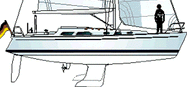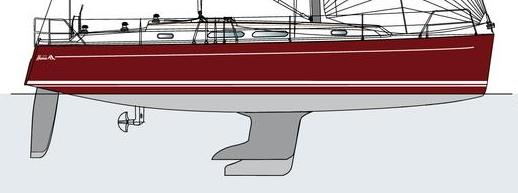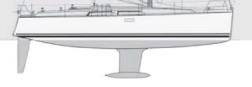##Hanse 37  Variants

From 1995 to 2005 the model was called the 371. In 2003 the freeboard was increased by about two inches.LWL = 32.32, Beam = 11.78, Draft = 6.49/5.55, Displ = 13120

I = 49.38, J = 14.14, P = 46.43 E = 15.16

In 2005, the 370 was introduced. In 2009 the 375 was introduced. The Hanse 370 and 375 have the same hull and rig. The major performance difference is in the keel.Hanse 370Hanse 375

LWL = 32.97, Beam = 12.30, Draft = 6.40/5.25 Displ = 14991/15873

I = 49.22, J = 13.62, P = 47.25, E = 16.90

.